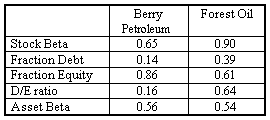### CFA Practice Question

There are 233 practice questions for this study session.

### CFA Practice Question

Assume GE is evaluating an investment in the oil and gas industry. GE would examine existing firms that are pure plays (public firms operating only in the oil and gas industry). Let's say GE selects Berry Petroleum & Forest Oil as pure plays:These firms are operationally similar, but Berry Petroleum's βE = 0.65 and Forest Oil's βE = 0.90. (Why are they so different? Forest Oil uses debt for 39% of financing; Berry Petroleum: 14%)

Assume:

• Risk-free rate of interest is 6%.
• Tax rate is 0%.
• The market's expected rate of return is 10%.
• GE's debt/equity ratio is 25%.
• Investors expect 6.5% on GE's bonds:

Using CAPM, compute the rate of return GE shareholders require for the oil and gas investment.

To determine the correct βA to use as discount rate for the project, GE must convert pure play βE to βA, then average.

Unlevered equity beta (which is the same as βA when taxes are zero) strips out the effect of financial leverage, so it is always less than or equal to equity beta.

Berry's βA = 0.56 and Forest's βA = 0.54, so average βA = 0.55.

GE's capital structure consists of 20% debt and 80% equity (D/E ratio = 0.25). Compute re-levered equity beta: βGE = βA (1 + D/E) = 0.55 (1 + 0.25) = 0.69.

The required rate of return on equity is 6% + 0.69 x (0.1 - 0.06) = 8.76%.

WACC = 20% x 6.5 + 80% x 8.76 = 8.308%

User Comment
taowu good one!
LloydBraun7 Don't we have to unlever the industry asset beta before we relever it? I took the industry beta, 0.55, and divided it by 1 + the average D/E, which is (0.64+0.16)/2.
0.55/1.4 = 0.3929.
Then I relevered by multiplying 0.3929 by 1.25 and my answer is 0.49.
reganbaha To re-lever the industry unlevered beta, in this case since tax rate = 0%, just multiply the beta by 1+D/E. = 0.55*1.25 = 0.69.
deepak50 If the debt / equity ratio is 25%, why use 20% and 80% for WACC?
deepak50 Oh ok I got it!
hoyleng deepak : D/E = 0.25

Assuming: D = 100; 100/E = 0.25
E = 100/0.25 = 400
Wd = 100/(100+400) =20% ;
We = 400/(100+400) = 80%
maria15 Where did they get the 6.5?
maria15 I just found it.
gulfa99 how can you answer this type of questions in 1.5 min during the exam?
arudkov u should answer it @60 sec. no more in order 2 feel yourself comfortable.
cfaajay why has he calculated WACC..was that needed ,in question the required return of shareholder's is asked..so is'nt required return on equity should be the answer .....WACC is less and as there is Debt in corporate structure of the firm ,the required return on equity should be higher..and answer should complete @ 8.76%..
johntan1979 I used the unlever, relever way, which I realized is longer. Just follow the way given in the answer. Same answer and faster.
johntan1979 And just to point out the similarity... you unlever and relever the stock beta to get the unlevered beta, which is the same as the asset beta.

Asset = Liabilities + SE
kirantailu How is required rate of return calculated. Not sure why relevered equity beta is multiplied by (0.1-0.06)
kirantailu got it
leon121 why isn't the stock beta used? I used the stock beta and used that to unlever it....
vadfir WACC -> cost of capital vs. CAPM -> cost of equity. Questions asks to compute rate of return GE shareholders require for oil and gas investment. Therefore, WACC calc is needed. Remember that WACC is cost to the firm for maintaining funds (debt +equity) provided by investors.
houstcarr 8.308% is the WACC which is not what shareholders require for a return, it is the effective cost of funding projects for a firm based on their target capital structure. If WACC and shareholders' required rate of return were the same thing, then what is CAPM for?...
rodney176 I'm reading shareholders and hearing cost of equity ..anyone else hear the same ?
sahilb7 Me too :(
jagp WACC = cost of capital to the firm (considering both funding sources, debt and equity). what shareholders require is the equity cost of capital (Re). That's why at some point, when you undertake to many projects using leverage, the equity cost of capital starts rising exponentially. For me, and with what the question ask, I would go for Re without giving it too much thought.
Akiva Isn't beta_A for Forest Oil 0.55 (not 0.54)?
See my calculations:
beta_E = beta_A * (1 + D / E)
beta_A = 0.90 / (1 + 0.64) = 0.90 / 1.64 = 0.5488
According to the rules of rounding 0.5488 is 0.55, so Forest's beta_A should be 0.55, not 0.54